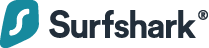# How to Get the Substring Between Two Characters in JavaScript

### Surfshark VPN: Your ultimate cyber shieldProtecting your connection is key. Surfing the web, you're exposed to many risks: data breaches, hacking attacks, & snoopers. One solution to fight them all — a VPN.

### 1. String substring(), indexOf() and lastIndexOf() Methods

To get the substring of a string between two of its characters in JavaScript, call the `slice()` method on the string, passing the index after the first occurrence of the first character as the first argument, and the index of the last occurrence of the second character as the second argument. For example:

``````function getSubstring(str, char1, char2) {
return str.substring(
str.indexOf(char1) + 1,
str.lastIndexOf(char2)
);
}

const str1 = 'one:two;three';
const substr1 = getSubstring(str1, ':', ';');
console.log(substr1); // two

const str2 = 'one?two!three';
const substr2 = getSubstring(str2, '?', '!');
console.log(substr2); // two
``````

The `String` `indexOf()` method returns the position of the first occurrence of a value in a string. On the other hand, `lastIndexOf()` returns the position of the last occurrence of a value in a string.

The `String` `substring()` method returns the portion of a string between the start and end indexes, specified by the first and second arguments respectively. We add `1` to the result of `indexOf()` because we don’t want the first character to be included in the substring that we’re trying to get. However, we don’t need to subtract `1` from the result of `lastIndexOf()`, because `substring()` already excludes the character at the specified end index.

If the value doesn’t exist in the string, both `indexOf()` and `lastIndexOf()` will return `-1`. This means that when the first character doesn’t exist in the string, all of the string from the start to the last occurrence of the second character will be included in the string.

``````const str1 = 'one:two;three';
const substr1 = getSubstring(str1, '-', ';');
console.log(substr1); // one:two
``````

Also, when the second character doesn’t exist, all of the string from the beginning to the first occurence of the first character will be included in the string.

``````const str1 = 'one:two;three';
const substr1 = getSubstring(str1, ':', '-');
console.log(substr1); // one``````

Depending on our use case, this might not be what we want. If we want an empty string (`''`) to be returned when either of the characters doesn’t exist, we’ll need to explicitly check for this:

``````function getSubstring(str, char1, char2) {
const char1Index = str.indexOf(char1);
const char2Index = str.lastIndexOf(char2);
if (char1Index === -1) return '';
if (char2Index === -1) return '';
return str.substring(char1Index, char2Index);
}

const str1 = 'one:two;three';
const substr1 = getSubstring(str1, '-', ';');
console.log(substr1); // '' (empty string)

const substr2 = getSubstring(str1, ':', '-');
console.log(substr2); // '' (empty string)
``````

### 2. String split(), Array slice(), and Array join() Methods

Here’s another way to get the substring of a string between two of its characters:

``````function getSubstring(str, char1, char2) {
return str
.split(char1)
.slice(1)
.join('')
.split(char2)
.slice(0, -1)
.join('');
}

const str1 = 'one:two;three';
const substr1 = getSubstring(str1, ':', ';');
console.log(substr1); // two

const str2 = 'one?two!three';
const substr2 = getSubstring(str2, '?', '!');
console.log(substr2); // two``````

The `String` `split()` method divides a string using a specified separator.

``````const str1 = 'one:two;three';

// [ 'one', 'two;three' ]
console.log(str1.split(':')); ``````

The `Array` `slice()` methods extracts the elements of an array between the start and end indexes, which are specified by the first and second arguments respectively. We pass `1` as the first argument without specifying a second, so `slice()` extracts from the element at index `1` to the end of the string.

``````// ['two;three'];
console.log([ 'one', 'two;three' ].slice(1));``````

We call the `Array` `join()` method on the result of `slice()` to concatenate the elements of the array into a string.

``````const str1 = 'one:two;three';

// two;three
console.log(['two;three'].join(''));
``````

We split this result again, this time by the second character.

``````// ['two', 'three'];
console.log('two;three'.split(';'));
``````

We call `slice()` on the array resulting from this split, passing `0` and `-1` as arguments, to copy all the array elements except the last one to a new array.

``````// [ 'two' ]
console.log(['two', 'three'].slice(0, -1));
``````

Finally, we call `join()` on this result to get the string between the two characters.

Unlike the first method, this approach handles the case where one of the characters is not in the string by returning an empty string.

``````function getSubstring(str, char1, char2) {
return str
.split(char1)
.slice(1)
.join('')
.split(char2)
.slice(0, -1)
.join('');
}

const str1 = 'one:two;three';
const substr1 = getSubstring(str1, '-', ';');
console.log(substr1); // '' (empty string)

const substr2 = getSubstring(str1, ':', '-');
console.log(substr2); // '' (empty string)
``````

## 11 Amazing New JavaScript Features in ES13

This guide will bring you up to speed with all the latest features added in ECMAScript 13. These powerful new features will modernize your JavaScript with shorter and more expressive code.